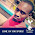## Arithmetic operators in c++ with example

Different Arithmetic operators used c are shown in table.

 Operator function * Multiplication / Division % Remainder + Addition - Subtraction

* operator is used give product of 2 numbers.

/ Operator gives Quotient after dividing Divisor and Dividend. Divisor should be nonzero number.

% operator gives remainder after division of 2 numbers. % operator can be used only for integer data type.

+ operator gives sum of 2 numbers.

- operator used to subtract one number from another number.

Note:-

# If both number are positive than answer will be in positive number.
# If either or both number are negative than answer will be either in positive or in negative, it is machine dependent.

Example:-

Program to find sum of 2 number.

Program to find product of 2 number.

Program to find subtract 2 number.

Program to find division 2 number.

Program to find remainder.

Previous Code:-

List Code:-
C++ Codes

Next Code:-
Logical Operators

C++ Programs
List of c++ Programs

### One Comment

1.